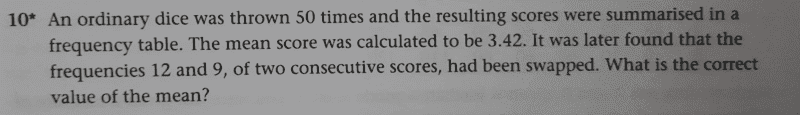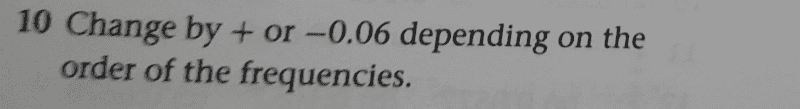# Find the correct value of the mean in the given problem

• chwala
In summary, the problem is that the author does not specify the correct value of the mean, and the solution is to find the mean using the three methods given.

#### chwala

Gold Member
Homework Statement
See attached.
Relevant Equations
Statistics
Find the problem and solution below;Now the part i do not seem to understand from the given solution is the negative value i.e to be specific ##-0.06##
In my understanding we shall have ##5## possibilities with each giving the correct mean value of ##3.48## which implies ##+0.06## from the given value on the text unless they meant ##3.48- 0.06=3.42##
They also did not indicate the correct value of the mean rather the difference.

My approach to the solution is as follows;

Consider the consecutive scores##1=12## and ##2=9## then it follows that the correct value of the mean will be given by;

##(171-30)+1(9)+2(12)=174##

##\dfrac{174}{50}=3.48##

Consider the consecutive scores##2=12## and ##3=9## then it follows that the correct value of the mean will be given by;

##(171-51)+2(9)+3(12)=174##

##\dfrac{174}{50}=3.48##

Consider the consecutive scores##3=12## and ##4=9## then it follows that the correct value of the mean will be given by;

##(171-72)+3(9)+4(12)=174##

##\dfrac{174}{50}=3.48##

Consider the consecutive scores##4=12## and ##5=9## then it follows that the correct value of the mean will be given by;

##(171-93)+4(9)+5(12)=174##

##\dfrac{174}{50}=3.48##

Consider the consecutive scores##5=12## and ##6=9## then it follows that the correct value of the mean will be given by;

##(171-114)+5(9)+6(12)=174##

##\dfrac{174}{50}=3.48##

Of course i would appreciate any other better approach. Cheers guys.

#### Attachments

What if, e.g, it says 5=9 and 6=12 and then you had to swap them (so the opposite direction of your post)

•chwala
You could have used a variable, n, in your method:
Suppose the incorrect calculation included Incorrect=12n + 9(n+1) = 21n+9. Then the correct calculation would be Correct=9n+12(n+1) = 21n+12. The difference (removing the Incorrect and adding the Correct) would be -(21n+9)+(21n+12) = 3. That would change the final mean of the 50 samples by 3/50 = 0.06.
Now suppose the opposite mistake was made. A similar calculation says that the difference would be -3, giving a change of -3/50=-0.06.

•chwala
Office_Shredder said:
What if, e.g, it says 5=9 and 6=12 and then you had to swap them (so the opposite direction of your post)
True, we shall have a mean value of ##3.36##Thanks.

FactChecker said:
You could have used a variable, n, in your method:
Suppose the incorrect calculation included Incorrect=12n + 9(n+1) = 21n+9. Then the correct calculation would be Correct=9n+12(n+1) = 21n+12. The difference (removing the Incorrect and adding the Correct) would be -(21n+9)+(21n+12) = 3. That would change the final mean of the 50 samples by 3/50 = 0.06.
Now suppose the opposite mistake was made. A similar calculation says that the difference would be -3, giving a change of -3/50=-0.06.
@FactChecker smart move there...